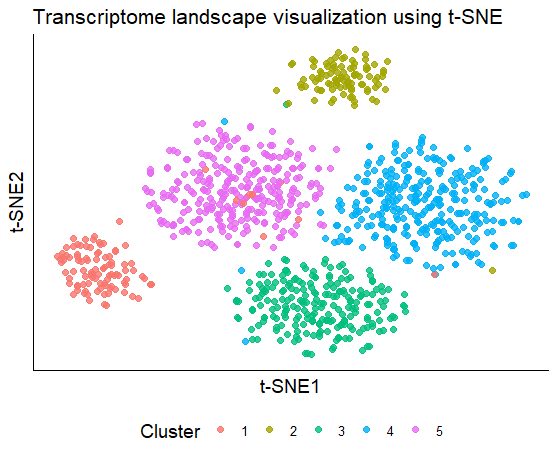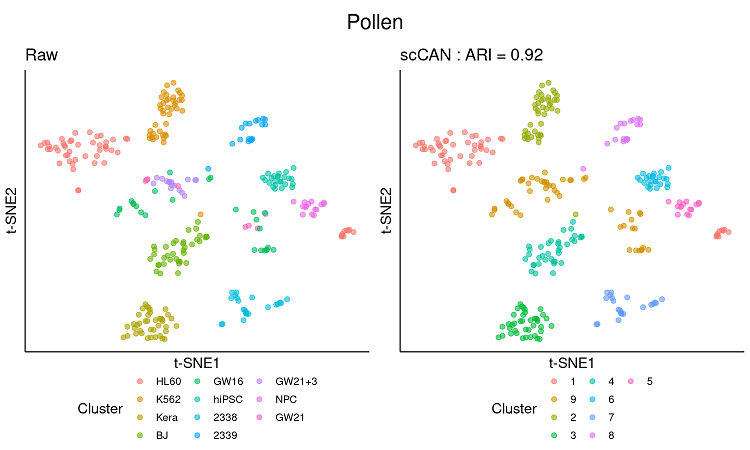# Introduction

Clustering is the key step to define cell types from the heterogeneous cell population. One critical challenge is that single-cell RNA sequencing (scRNA-seq) experiments generate a massive amount of data with an excessive noise level, which hinders many computational algorithms. We propose a single cell Clustering method using Autoencoder and Network fusion (scCAN) that can accurately segregate cells from the high-dimensional noisy scRNA-Seq data.

# Preliminaries

This vignette provides step-by-step instruction for scCAN R package installation, analyses using example and scRNA-seq datasets, and an in-depth analysis using scRNA-seq data with prior biological knowledge.

Install scCAN package from CRAN repository.

``install.packages("scCAN")``

To start the analysis, load scCAN package:

``library(scCAN)``
``## Loading required package: scDHA``
``## Loading required package: FNN``
``## Loading required package: purrr``

# Analysis on the example dataset

The input of scCAN is an expression matrix in which rows represent samples and columns represent genes. scCAN package includes an example dataset of 1,000 cells and 2,000 genes.

``````#Load example data (SCE dataset)
data("SCE")
#Get data matrix and label
data <- t(SCE\$data); label <- as.character(SCE\$cell_type1)``````

Inspect the example of 10 cells and 10 genes.

``data[1:10,1:10]``
``````##        [,1] [,2] [,3] [,4] [,5] [,6] [,7] [,8] [,9] [,10]
## Cell1    41   57   45   12    0    0    6    6    1   129
## Cell2    73   55   45    3    8    0   30   33    0   176
## Cell3    46   62   40    3   11    0    5   21    0   106
## Cell4    37   39   13    0   16    0    5    0    0    61
## Cell5    77   76   72    0   18    0   10   39    4   140
## Cell6    23   34   16    0    0    0    5   21    0    62
## Cell7     0   15   11    0    0    0    6   10    0    31
## Cell8    58   56   63    8    9    0    4   20    0   112
## Cell9    24   20   34    1    0    0    2   38    0    46
## Cell10   71   61   61    8   14    0   20   23    0     0``````
``dim(data)``
``##  1000 2000``

Next, we can cluster the example dataset using the following command.

``````#Generate clustering result. The input matrix has rows as samples and columns as genes
result <- scCAN(data)
#The clustering result can be found here
cluster <- result\$cluster``````

Users can visualize the expression data with the new cluster annotation. To reduce the dimension, users can use the package irlba  to obtain the first 20 principal components, and the package Rtsne  to obtain the transcriptome landscape using t-SNE. Finally, we can visualize the cell landscape using scatter plot available in ggplot2  package.

``````suppressPackageStartupMessages({
library(irlba)
library(ggplot2)
library(Rtsne)
})
# Get 2D emebedding data of the original data.
set.seed(1)
low <- irlba::irlba(data, nv = 20)\$u
tsne <- as.data.frame(Rtsne::Rtsne(low)\$Y)
tsne\$cluster <- as.character(cluster)
colnames(tsne) <- c("t-SNE1","t-SNE2","Cluster")
p <- ggplot2::ggplot(tsne, aes(x = `t-SNE1`, y = `t-SNE2`, colour = `Cluster`))+
ggtitle("Transcriptome landscape visualization using t-SNE")+labs(color='Cluster') +
geom_point(size=2, alpha = 0.8) +
theme_classic()+
theme(axis.text.x=element_blank(),
axis.ticks.x=element_blank(),
axis.text.y=element_blank(),
axis.ticks.y=element_blank(),
axis.title=element_text(size=14),
plot.title = element_text(size=16),
panel.grid.major = element_blank(),
panel.grid.minor = element_blank(),
legend.position="bottom", legend.box = "horizontal",
legend.text =element_text(size=10),
legend.title=element_text(size=14))+
guides(colour=guide_legend(nrow = 1))
p``````Visualization of expression data using cells clusters identified from scCAN.

We will use a real dataset from Pollen et al. that is available on our website. This dataset has 301 cells sequenced from human tissues including blood, skin, brain, and stem cells.

``````suppressPackageStartupMessages({
library(SummarizedExperiment)
})
path <- "https://bioinformatics.cse.unr.edu/software/scCAN/data/pollen.rds"
# Get expression matrix
data <- t(SummarizedExperiment::assay(SCE))
# Get cell annotation
label <- SummarizedExperiment::colData(SCE)``````

Next, we can cluster dataset using the following command.

``````#Generate clustering result, the input matrix has rows as samples and columns as genes
start <- Sys.time()
result <- scCAN(data)
running_time <- difftime(Sys.time(),start,units = "mins")
print(paste0("Running time = ", running_time))
#The clustering result can be found here
cluster <- result\$cluster``````
``## Running time = 1.293936 mins``

This dataset also has an annotation file that includes true cell labels:

``head(label)``
``````DataFrame with 6 rows and 2 columns
cell_type1 cell_type2
<factor>   <factor>
Hi_2338_1       2338     dermal
Hi_2338_2       2338     dermal
Hi_2338_3       2338     dermal
Hi_2338_4       2338     dermal
Hi_2338_5       2338     dermal
Hi_2338_6       2338     dermal``````

The annotation file is stored data frame in which rows are samples. The annotation file has two sets of labels: (i) cell_type1 indicates cell types discovered from Pollen et al., (ii) cell_type2 has annotation of tissues that the cells was taken. Users can compare the obtain clustering results against cell_type1 for validation and visualization.

``````#Calculate adjusted Rand Index using mclust package
print(paste0("ARI = ", ari))``````

Users can use the same code above to plot the transcriptome landscape of the Pollen dataset. Below is the transcriptome landscapes of the dataset using the annotation provided by Pollen et al., and the landscape using the new cluster annotation.Visualization of expression data using original cell types and cluster identified from scCAN for Pollen dataset.

scCAN was able to cluster Pollen dataset in 1 minutes with 9 clusters identified.The clusters discovered by scCAN are highly correlated with cell labels obtained from the original publication  with an ARI of 0.92.

## Visualizing the data using latent variables of scCAN

scCAN generates a compressed representation of original expression data. This representation is part of the output, and can be obtained using the following code:

``````suppressPackageStartupMessages({
library(ggplot2)
library(cowplot)
library(scatterplot3d)
library(scales)
})
# Get latent data from scCAN result
latent <- result\$latent
# Generating 2D embedding data using 2 first latent variables
data1= latent[,1:2]

# Generating 3D embedding data using 3 first latent variables
data2= latent[,1:3]

#Plotting 2D data using ggplot
name ="Pollen"
dat <- as.data.frame(data1)
dat\$p <- as.character(cluster)
colnames(dat) <- c("Latent Variable 1", "Latent Variable 2", "scCAN Cluster")
p1 <- ggplot(dat, aes(x = `Latent Variable 1`,y = `Latent Variable 2`,colour = `scCAN Cluster`)) +
theme(plot.title = element_text(hjust = 0.5)) +
geom_point(size=2, alpha = 0.6) +
#scale_color_manual(values = colors)+
theme_classic()+
theme(axis.text.x=element_blank(),
axis.ticks.x=element_blank(),
axis.text.y=element_blank(),
axis.ticks.y=element_blank(),
axis.title=element_text(size=14),
panel.grid.major = element_blank(),
panel.grid.minor = element_blank(),
legend.position="none", legend.box = "horizontal",
legend.text =element_text(size=12),
legend.title=element_text(size=14) )+
guides(colour=guide_legend(ncol=2))
title1 <- ggdraw() + draw_label(name, size=16)
legend1 <- get_legend(p1 +
theme(legend.position="bottom", legend.box = "horizontal",legend.title=element_text(size=12),
legend.margin = margin(0), legend.spacing.x = unit(0.1, "cm") ,
legend.text=element_text(size=12)) + guides(color=guide_legend(nrow = 2))
)

m1 <- plot_grid(title1, p1, legend1, rel_heights = c(0.05, 0.5, .1), ncol = 1)
plot_grid(m1,rel_heights = c(0.05, 0.9), ncol = 1)

#Plotting 3D data using scatterplot3d package
graphics.off()
name = "Pollen"
dat <- as.data.frame(data2)
# dat\$t <- label\$cell_type1
dat\$p <- as.character(cluster)
colnames(dat) <- c("Latent Variable 1", "Latent Variable 2", "Latent Variable 3", "scCAN Cluster")
pal <- hue_pal()(length(unique(cluster)))
colors <- pal[as.numeric(dat\$`scCAN Cluster`)]

scatterplot3d(dat[,1:3], pch = 16,
grid=TRUE, box=TRUE,color = colors)``````

The 2D and 3D visualizations using 2 latent variables and 3 latent variables obtained from latent data are shown as follows: